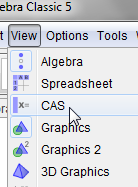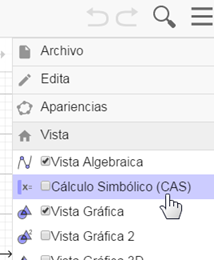# Intersection point disappearing

SharkD shared this question 2 years ago

In the attachment, points A and B have identical definitions, yet one is visible and the other is undefined. This happens often in my larger worksheet when I move the sliders in the top right of the screen. Is there a workaround? Thanks.1

If someone knows a way to calculate the coordinates of these points (thereby not needing the intersection) that would be okay as well! Thanks!1

try redefining P_1 as P_{1}

in some version there was a problem with subscript names1

No luck. :(

I've tried to do as you say. There are only two points now, A and B. If you move the slider "sphyaw1" the points A and B will disappear at different times despite sharing the same definition.1

my english is not enough for explaning my ideas. I hope google translator do a good translation

siento no poder responder satisfactoriamente a tu pregunta porque los metodos numericos no siempre son igual de precisos incluso cuando aparentemente la definicion parezca la misma. de hecho las definiciones en el momento en que A es mostrado y B es indefinido no son la misma. si pones el redondeo en 13 decimales podrá observar dicha diferencia en la definicion pues GG va modificando el punto de comienzo del calculo numerico en cada movimiento del deslizador y una pequeña diferencia en el punto de comienzo puede dar en algunos casos una gran diferencia en el punto calculado o en las condiciones en que dicho punto es encontrado o no

en el adjunto puede ver que en realidad la definicion de A y de B son diferentes en el punto inicial

puede intentar dar un punto inicial igual en ambos puntos pero eso no creo que asegure que GG lo mantenga a lo largo del proceso1

Thank you. I did not think to increase the rounding. So simple. :(1

If you define the objects as conics (not parametric curves) then it should work better1

Yes, I tried that in the past and you are correct. However, then I end up with two copies of everything. (Parametric and conic.) Such a mess! Plotting the points using equations instead of intersections would work too, but I do not know these equations.1

So, I added some conics to serve as replacements for the parametric curves. However, in the attachment I try to create intersections between the conics b_2 and a_0, but geogebra creates three intersection points instead of two. Two of the points are identical even when rounding to 15 places. I am unable to delete just one of these, as trying to delete just one ends up deleting them all. Is there a way to prevent these issues? Thanks.1

Hi, If the problem is only in visibility, replace the intersection points with a list l1={Intersect(b_2, a_0)}. l11 = Unique(l1)1

Thank you. It needs to be more than just visual however.1

suppose you have F_{1}=Curve(cos(30°) sin(phi) a11 + cos(phi) a12 + sin(30°) sin(phi) a13, cos(30°) sin(phi) a21 + cos(phi) a22 + sin(30°) sin(phi) a23, phi, 0°, 180°)

but you want define F_{1} in CAS

you write Curve(cos(30°) sin(phi) a11 + cos(phi) a12 + sin(30°) sin(phi) a13, cos(30°) sin(phi) a21 + cos(phi) a22 + sin(30°) sin(phi) a23, phi, 0°, 180°) in CAS and you get ......................surprise!!!!!! A conic!

it is easy reduce the equations to cte cos(phy)=expr1(x,y) and cte' sin(phy)=expr2(x,y) then to get the conic. The CAS do the job for us

or you can create F_{1}(10º) F_{1}(30º) F_{1}(50º) F_{1}(70º) F_{1}(90º) and the conic through five points1

Sorry, but what is CAS?

`or you can create F_{1}(10º) F_{1}(30º) F_{1}(50º) F_{1}(70º) F_{1}(90º) and the conic through five points`

This is what I ended up doing.1

`Sorry, but what is CAS? `1

Thanks!! I just tested this and I think it ALSO resolves the problem of there being too many intersection points. Are there any potential disadvantages to using CAS? One thing I have noticed is that I don't know how to change the sort order of CAS items. Can I move them up/down in the list?1

sorry, no1

I can't rename the CAS curves either, it seems. This ends up becoming impractical because I have many such curves.1

"Thanks!! I just tested this and I think it ALSO resolves the problem of there being too many intersection points."

I take that back. I have seen the same issues with the CAS conics too. I think this cannot be solved as long as the Curve command is used at some stage, period.

I will continue to look out for a method of plotting the points using an equation instead of the intersect command.